# Mirrors and LensesPage 2

#### WATCH ALL SLIDES

This is called the mirror equation

Slide 8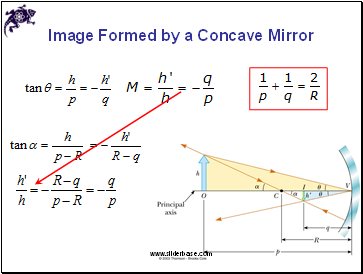Image Formed by a Concave Mirror

Slide 9## Focal Length

If an object is very far away, then p =  and 1/p = 0

Incoming rays are essentially parallel

In this special case, the image point is called the focal point

The distance from the mirror to the focal point (f) is called the focal length

The focal point is dependent solely on the curvature of the mirror, not by the location of the object

Slide 10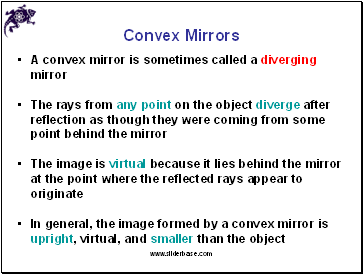## Convex Mirrors

A convex mirror is sometimes called a diverging mirror

The rays from any point on the object diverge after reflection as though they were coming from some point behind the mirror

The image is virtual because it lies behind the mirror at the point where the reflected rays appear to originate

In general, the image formed by a convex mirror is upright, virtual, and smaller than the object

Slide 11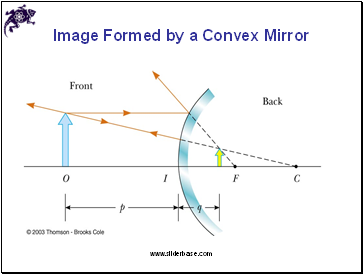Image Formed by a Convex Mirror

Slide 12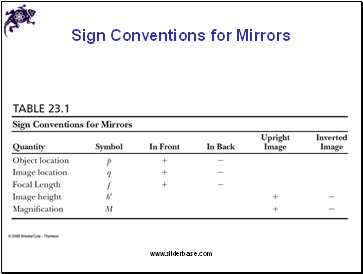Sign Conventions for Mirrors

Slide 13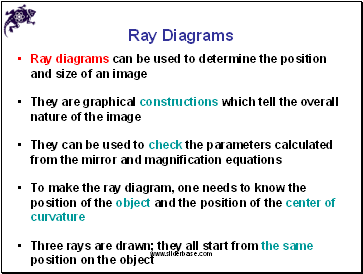## Ray Diagrams

Ray diagrams can be used to determine the position and size of an image

They are graphical constructions which tell the overall nature of the image

They can be used to check the parameters calculated from the mirror and magnification equations

To make the ray diagram, one needs to know the position of the object and the position of the center of curvature

Three rays are drawn; they all start from the same position on the object

Slide 14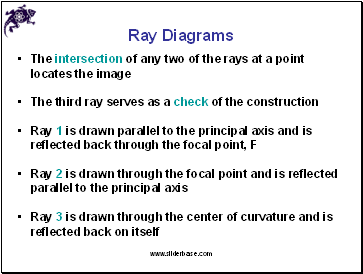Ray Diagrams

The intersection of any two of the rays at a point locates the image

The third ray serves as a check of the construction

Ray 1 is drawn parallel to the principal axis and is reflected back through the focal point, F

Ray 2 is drawn through the focal point and is reflected parallel to the principal axis

Ray 3 is drawn through the center of curvature and is reflected back on itself

Slide 15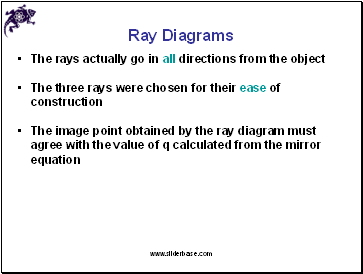Ray Diagrams

The rays actually go in all directions from the object

The three rays were chosen for their ease of construction

The image point obtained by the ray diagram must agree with the value of q calculated from the mirror equation

Slide 16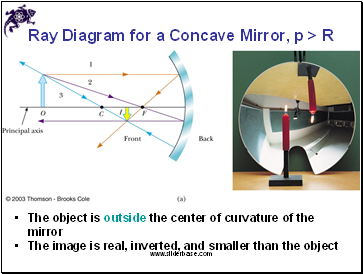## Ray Diagram for a Concave Mirror, p > R

Go to page:
1  2  3  4  5  6  7